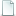## [Coursera] Introduction to Mathematical Thinking Dr. Keith Devlin (Stanford University)coursera-intro-to-maths-thinking (44 files)assignments/Assignment_1.pdf 81.18kBassignments/Assignment_10_1.pdf 62.36kBassignments/Assignment_10_2.pdf 76.96kBassignments/Assignment_2.pdf 68.32kBassignments/Assignment_3.pdf 53.83kBassignments/Assignment_4.pdf 93.35kBassignments/Assignment_5.pdf 73.68kBassignments/Assignment_6.pdf 64.05kBassignments/Assignment_7.pdf 79.78kBassignments/Assignment_8.pdf 77.07kBassignments/Assignment_9.pdf 64.79kBassignments/Assignment_9_SOLUTIONS.pdf 77.14kBassignments/Background_Reading.pdf 192.29kBassignments/Final_Exam.pdf 95.37kBassignments/Supplement_(Set_Theory).pdf 192.06kBlectures/supplementary_videos/Introduction to Mathematical Thinking 0.0 Meet the Team (710).mp4 30.12MBlectures/supplementary_videos/Introduction to Mathematical Thinking 0.1 Team Discussion (812).mp4 34.89MBlectures/week1/Introduction to Mathematical Thinking 1.0 Lecture 0 - Welcome (921).mp4 19.42MBlectures/week1/Introduction to Mathematical Thinking 1.1 Lecture 1 - Introductory Material (2919).mp4 68.03MBlectures/week1/Introduction to Mathematical Thinking 1.2 Lecture 2 - Logical Combinators (2755).mp4 75.81MBlectures/week2/Introduction to Mathematical Thinking 2.0 Lecture 3 - Implication (3138).mp4 93.15MBlectures/week2/Introduction to Mathematical Thinking 2.1 Lecture 4 - Equivalence (2427).mp4 67.31MBlectures/week2tuts/Introduction to Mathematical Thinking 3.0 Assignment 1 (212).mp4 6.21MBlectures/week2tuts/Introduction to Mathematical Thinking 3.1 Assignment 2 (1313).mp4 40.96MBlectures/week2tuts/Introduction to Mathematical Thinking 3.2 Problem Set 1 (941).mp4 29.29MBlectures/week3/Introduction to Mathematical Thinking 4.0 Lecture 5 - Quantifiers (2931).mp4 81.02MBlectures/week3/Introduction to Mathematical Thinking 4.1 Lecture 6 - Working with Quantifiers (3736).mp4 114.56MBlectures/week3tuts/Introduction to Mathematical Thinking 5.0 Assignment 3 (1105).mp4 19.26MBlectures/week3tuts/Introduction to Mathematical Thinking 5.1 Assignment 4 (2106).mp4 61.52MBlectures/week3tuts/Introduction to Mathematical Thinking 5.2 Problem Set 2 (2335).mp4 68.34MBlectures/week4/Introduction to Mathematical Thinking 6.0 Lecture 7 - Proofs (4657).mp4 128.16MBlectures/week4/Introduction to Mathematical Thinking 6.1 Lecture 8 - Proofs Involving Quantifiers (5056).mp4 156.59MBlectures/week4tuts/Introduction to Mathematical Thinking 7.0 Assignment 5 (4122).mp4 113.01MBlectures/week4tuts/Introduction to Mathematical Thinking 7.1 Assignment 6 (1638).mp4 47.19MBlectures/week4tuts/Introduction to Mathematical Thinking 7.2 Problem Set 3 (1045).mp4 29.78MBlectures/week5/Introduction to Mathematical Thinking 8.0 Lecture 9 - Number Theory (4711).mp4 140.55MBlectures/week5/Introduction to Mathematical Thinking 8.1 Lecture 10A - Real Analysis I (2726).mp4 77.81MBlectures/week5/Introduction to Mathematical Thinking 8.2 Lecture 10B - Real Analysis II (4631).mp4 141.79MBlectures/week5tuts/Introduction to Mathematical Thinking 9.0 Assignment 7 (1500).mp4 44.30MBlectures/week5tuts/Introduction to Mathematical Thinking 9.1 Assignment 8 (1608).mp4 48.14MBlectures/week5tuts/Introduction to Mathematical Thinking 9.2 Problem Set 4 (2440).mp4 75.34MBlectures/week6tuts/Introduction to Mathematical Thinking 10.0 Assignment 10 (1201).mp4 34.79MBlectures/week6tuts/Introduction to Mathematical Thinking 10.1 Problem Set 5 (1724).mp4 54.05MBREADME.txt 0.10kB
Type: Course
Tags:

Bibtex:
```@article{,
title= {[Coursera] Introduction to Mathematical Thinking},
keywords= {},
journal= {},
author= {Dr. Keith Devlin (Stanford University)},
year= {2015},
url= {},
abstract= {About this course: Learn how to think the way mathematicians do - a powerful cognitive process developed over thousands of years.

The goal of the course is to help you develop a valuable mental ability – a powerful way of thinking that our ancestors have developed over three thousand years.

Mathematical thinking is not the same as doing mathematics – at least not as mathematics is typically presented in our school system. School math typically focuses on learning procedures to solve highly stereotyped problems. Professional mathematicians think a certain way to solve real problems, problems that can arise from the everyday world, or from science, or from within mathematics itself. The key to success in school math is to learn to think inside-the-box. In contrast, a key feature of mathematical thinking is thinking outside-the-box – a valuable ability in today’s world. This course helps to develop that crucial way of thinking.

The course is offered in two versions. The eight-week-long Basic Course is designed for people who want to develop or improve mathematics-based, analytic thinking for professional or general life purposes. The ten-week-long Extended Course is aimed primarily at first-year students at college or university who are thinking of majoring in mathematics or a mathematically-dependent subject, or high school seniors who have such a college career in mind. The final two weeks are more intensive and require more mathematical background than the Basic Course. There is no need to make a formal election between the two. Simply skip or drop out of the final two weeks if you decide you want to complete only the Basic Course.

},
superseded= {},
terms= {}
}

```

Send Feedback